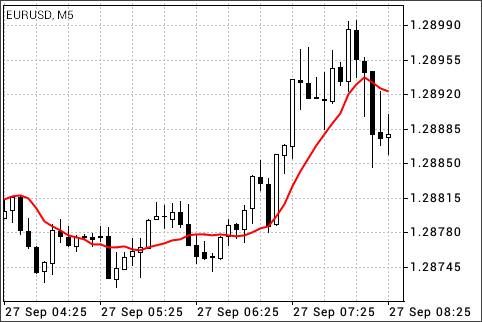MetaTrader 5 Android 帮助图表指标趋势指标移动均线 (Moving Average)

# 移动均线 (Moving Average)

• 简单移动平均线 (SMA)
• 指数移动平均线 (EMA)
• 平滑移动平均线 (SMMA)
• 线形加权移动平均线 (LWMA)## 计算

### 简单移动平均线 (SMA) #

SMA = SUM (CLOSE (i), N) / N

SUM ― 总和;
CLOSE (i) ― 当前周期收盘价;
N ― 计算所需的周期数。

### 指数移动平均线 (EMA) #

EMA = (CLOSE (i) * P) + (EMA (i - 1) * (1 - P))

CLOSE (i) ― 当前周期收盘价;
EMA (i - 1) ― 前一周期的移动均值;
P ― 使用价格的百分比。

### 平滑移动平均线 (SMMA) #

SUM1 = SUM (CLOSE (i), N)

SMMA1 = SUM1 / N

SMMA (i) = (SMMA1*(N-1) + CLOSE (i)) / N

PREVSUM = SMMA (i - 1) * N

SMMA (i) = (PREVSUM - SMMA (i - 1) + CLOSE (i)) / N

SUM ― 合计;
SUM1 ― 自前一根柱线开始的 N 周期收盘价总和;
PREVSUM ― 前一根柱线的平滑值合计;
SMMA (i-1) ― 前一根柱线的移动均线平滑值;
SMMA (i) ― 当前柱线的移动均线平滑值 (排除首根柱线);
CLOSE (i) ― 当前收盘价;
N ― 平滑周期。

SMMA (i) = (SMMA (i - 1) * (N - 1) + CLOSE (i)) / N

### 线形加权移动平均线 (LWMA) #

LWMA = SUM (CLOSE (i) * i, N) / SUM (i, N)

SUM ― 合计;
CLOSE(i) ― 当前收盘价;
SUM (i, N) ― 权重系数总和;
N ― 平滑周期。